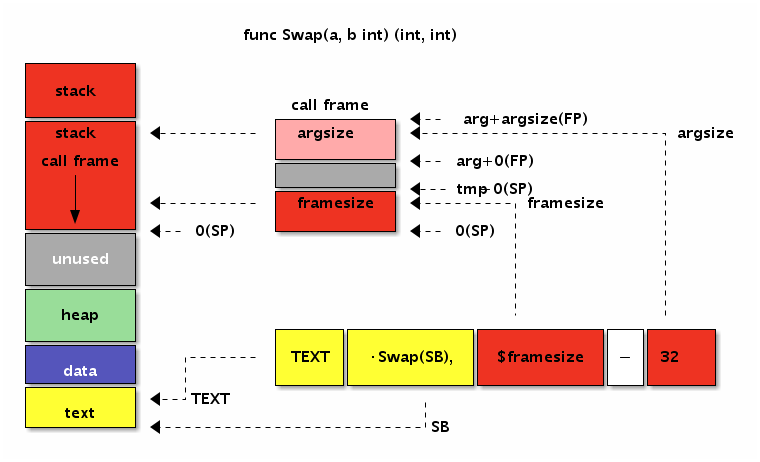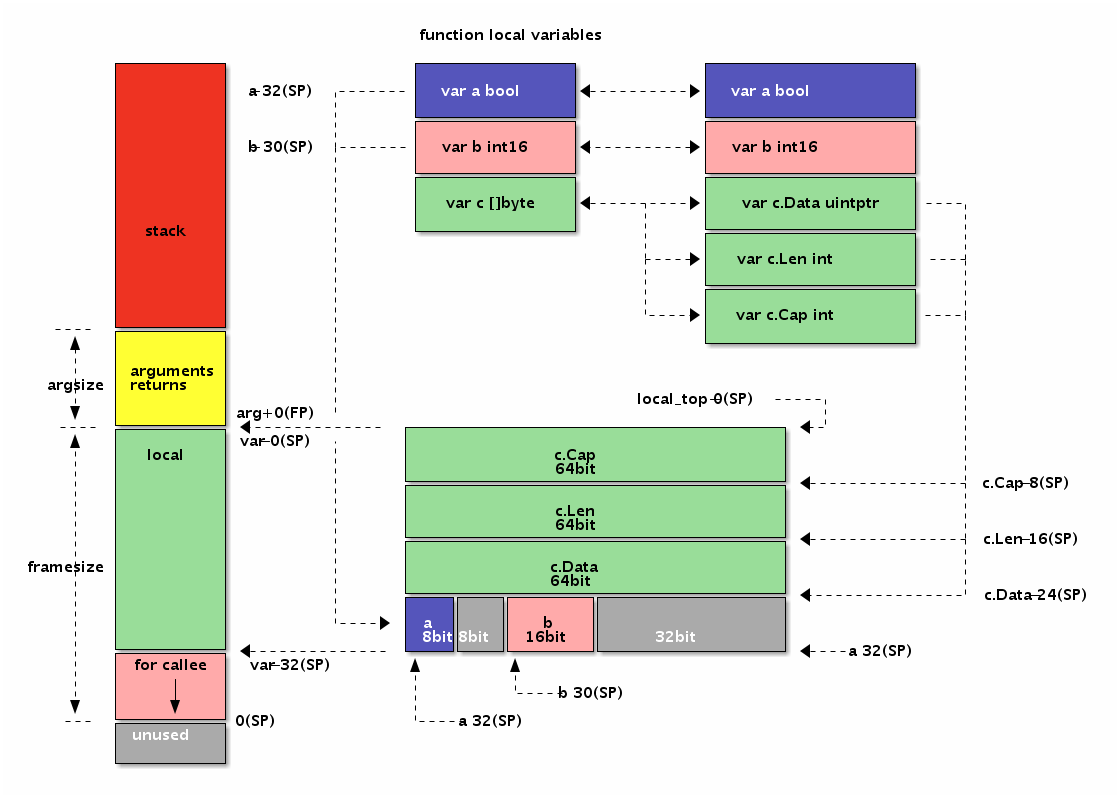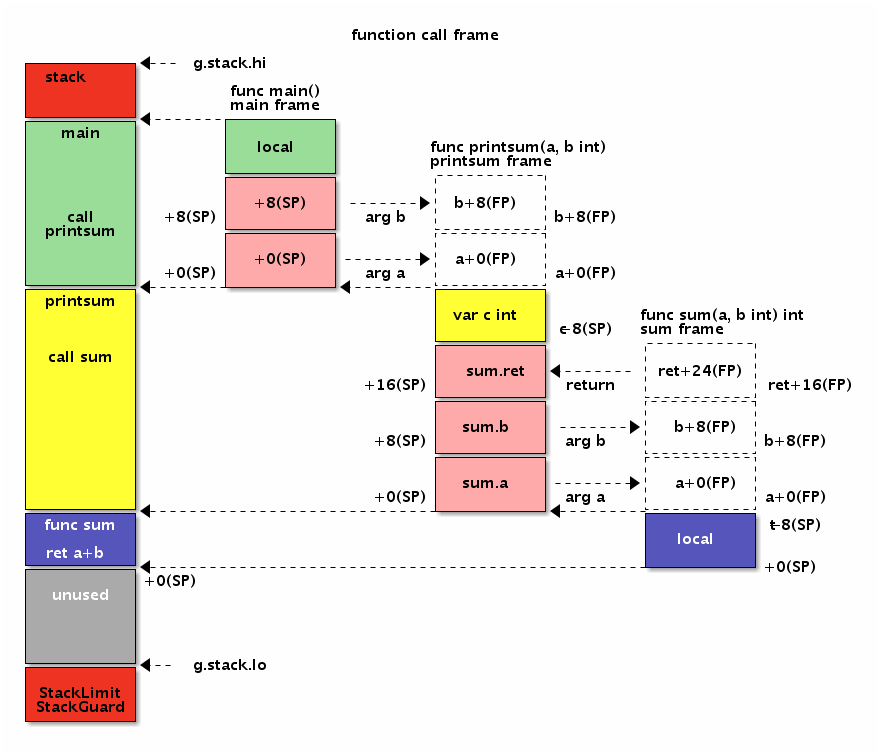# 3.4 函数

## 3.4.1 基本语法

``````TEXT symbol(SB), [flags,] \$framesize[-argsize]
``````

``````package main

//go:nosplit
func Swap(a, b int) (int, int)
``````

``````// func Swap(a, b int) (int, int)
TEXT ·Swap(SB), NOSPLIT, \$0-32

// func Swap(a, b int) (int, int)
TEXT ·Swap(SB), NOSPLIT, \$0
````````````func Swap(a, b, c int) int
func Swap(a, b, c, d int)
func Swap() (a, b, c, d int)
func Swap() (a []int, d int)
// ...
``````

## 3.4.2 函数参数和返回值

``````func Swap(a, b int) (ret0, ret1 int)
``````

``````TEXT ·Swap(SB), \$0-32
````````````TEXT ·Swap(SB), \$0
MOVQ a+0(FP), AX     // AX = a
MOVQ b+8(FP), BX     // BX = b
MOVQ BX, ret0+16(FP) // ret0 = BX
MOVQ AX, ret1+24(FP) // ret1 = AX
RET
``````

## 3.4.3 参数和返回值的内存布局

``````func Foo(a bool, b int16) (c []byte)
``````

``````type Foo_args struct {
a bool
b int16
c []byte
}
type Foo_returns struct {
c []byte
}
``````

``````func Foo(FP *SomeFunc_args, FP_ret *SomeFunc_returns) {
// a = FP + offsetof(&args.a)
_ = unsafe.Offsetof(FP.a) + uintptr(FP) // a
// b = FP + offsetof(&args.b)

// argsize = sizeof(args)
argsize = unsafe.Offsetof(FP)

// c = FP + argsize + offsetof(&return.c)
_ = uintptr(FP) + argsize + unsafe.Offsetof(FP_ret.c)

// framesize = sizeof(args) + sizeof(returns)
_ = unsafe.Offsetof(FP) + unsafe.Offsetof(FP_ret)

return
}
``````

Foo函数的参数和返回值的大小和内存布局：``````TEXT ·Foo(SB), \$0
MOVEQ a+0(FP),       AX // a
MOVEQ b+2(FP),       BX // b
MOVEQ c_dat+8*1(FP), CX // c.Data
MOVEQ c_len+8*2(FP), DX // c.Len
MOVEQ c_cap+8*3(FP), DI // c.Cap
RET
``````

## 3.4.4 函数中的局部变量

``````func Foo() {
var c []byte
var b int16
var a bool
}
``````

``````TEXT ·Foo(SB), \$32-0
MOVQ a-32(SP),      AX // a
MOVQ b-30(SP),      BX // b
MOVQ c_data-24(SP), CX // c.Data
MOVQ c_len-16(SP),  DX // c.Len
MOVQ c_cap-8(SP),   DI // c.Cap
RET
``````

Foo函数有3个局部变量，但是没有调用其它的函数，因为对齐和填充的问题导致函数的栈帧大小为32个字节。因为Foo函数没有参数和返回值，因此参数和返回值大小为0个字节，当然这个部分可以省略不写。而局部变量中先定义的变量c离伪SP寄存器对应的地址最远，最后定义的变量a离伪SP寄存器最近。有两个因素导致出现这种逆序的结果：一个从Go语言函数角度理解，先定义的c变量地址要比后定义的变量的地址更小；另一个是伪SP寄存器对应栈帧的底部，而X86中栈是从高向地生长的，所以最先定义有着更小地址的c变量离栈的底部伪SP更远。

``````func Foo() {
var local struct{
a bool
b int16
c []byte
}
var SP = &local;

_ = -(unsafe.Sizeof(local)-unsafe.Offsetof(local.a)) + uintptr(&SP) // a
_ = -(unsafe.Sizeof(local)-unsafe.Offsetof(local.b)) + uintptr(&SP) // b
_ = -(unsafe.Sizeof(local)-unsafe.Offsetof(local.c)) + uintptr(&SP) // c
}
``````## 3.4.5 调用其它函数

``````func main() {
printsum(1, 2)
}

func printsum(a, b int) {
var ret = sum(a, b)
println(ret)
}

func sum(a, b int) int {
return a+b
}
``````Go语言中函数调用是一个复杂的问题，因为Go函数不仅仅要了解函数调用参数的布局，还会涉及到栈的跳转，栈上局部变量的生命周期管理。本节只是简单了解函数调用参数的布局规则，在后续的章节中会更详细的讨论函数的细节。

## 3.4.6 宏函数

``````#define SWAP(x, y) do{ int t = x; x = y; y = t; }while(0)
``````

``````#define SWAP(x, y, t) MOVQ x, t; MOVQ y, x; MOVQ t, y
``````

``````// func Swap(a, b int) (int, int)
TEXT ·Swap(SB), \$0-32
MOVQ a+0(FP), AX // AX = a
MOVQ b+8(FP), BX // BX = b

SWAP(AX, BX, CX)     // AX, BX = b, a

MOVQ AX, ret0+16(FP) // return
MOVQ BX, ret1+24(FP) //
RET
``````